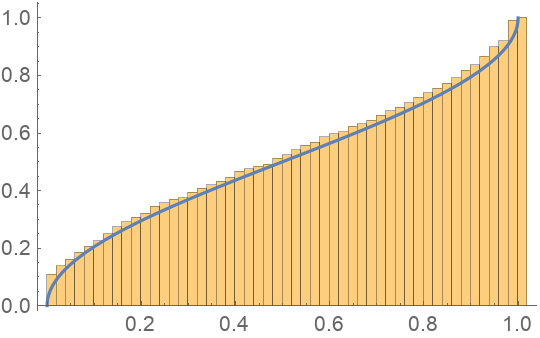#Function Repository Resource:

# ArcsineLawRandomnessTest

Use the arcsine law to assess the randomness of a sequence of zeros and ones

Contributed by: Emmy/Noah Blumenthal
noahb320@gmail.com
emmyb320@bu.edu
 ResourceFunction["ArcsineLawRandomnessTest"][sequence] uses the arcsine law to test sequence for randomness and returns an associated p–value. ResourceFunction["ArcsineLawRandomnessTest"][sequence,"property"] tests sequence for randomness and returns the specified property.

## Details and Options

Properties include:
 "TestStatistic" returns the test statistic "PValue" returns the p-value associated with the test
The test works only for sequences of zeros and ones.
Results are generally invalid for sequence lengths short than 100.
The test returns a two–tailed p–value.

## Examples

### Basic Examples

Generate a sequence of random integers and apply a runs-based test:

 In:=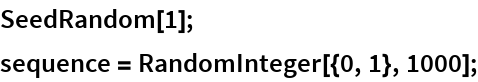Visualize the sequence:

 In:=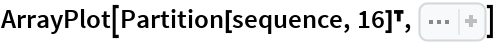Out=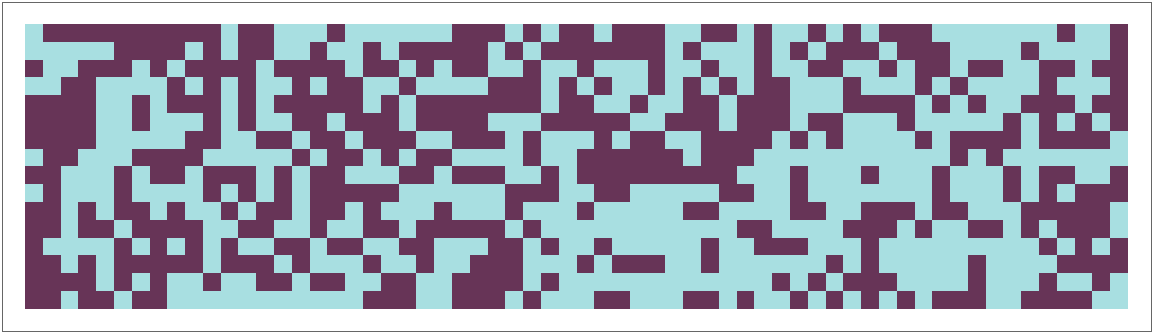In:=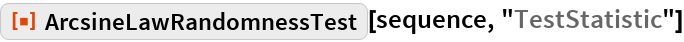Out=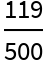In:=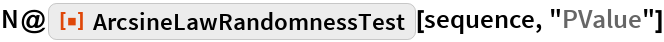Out=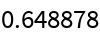### Applications

Reject a non-random sequence:

 In:=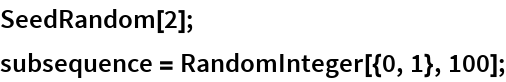In:=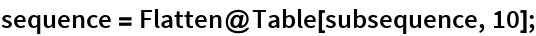In:=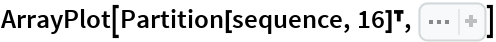Out=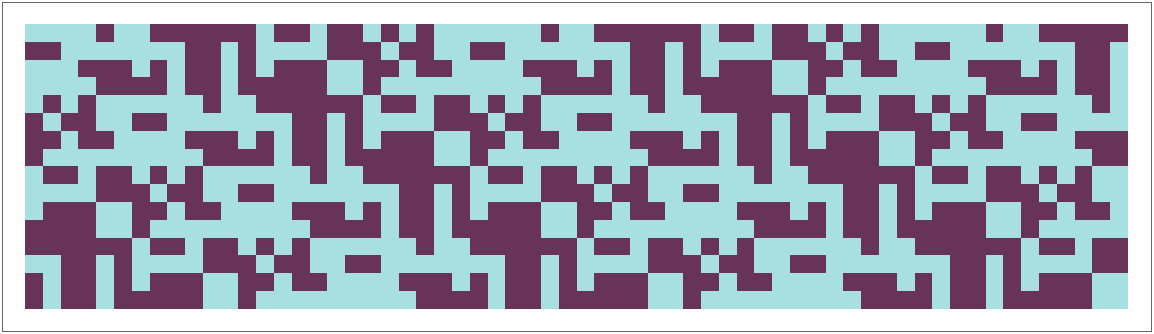In:=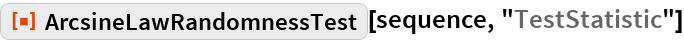Out=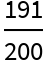In:=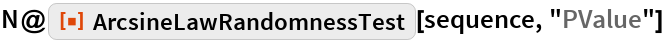Out=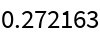Test the randomness of rule 30:

 In:=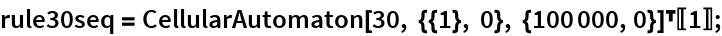In:=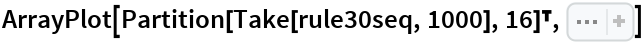Out=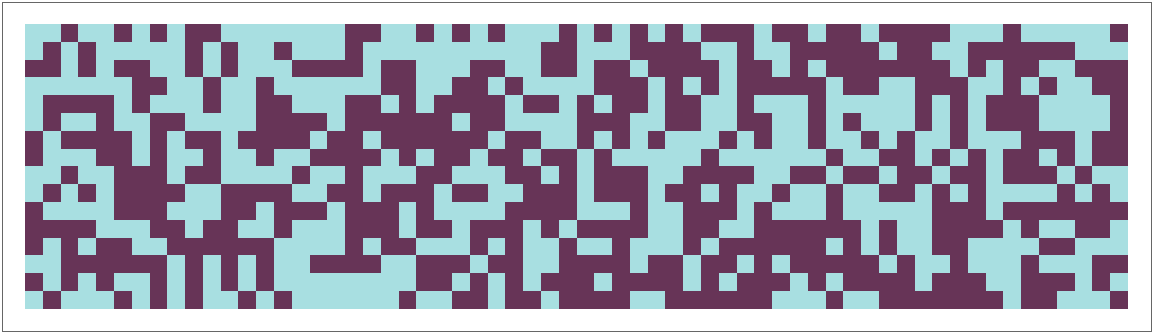In:=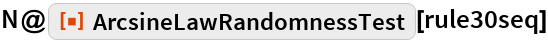Out=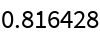### Possible Issues

ArcsineLawRandomnessTest requires sequences of length 100 or more:

 In:=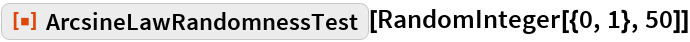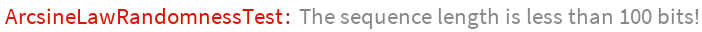### Neat Examples

Visualize the sampling distribution of the test statistic:

 In:=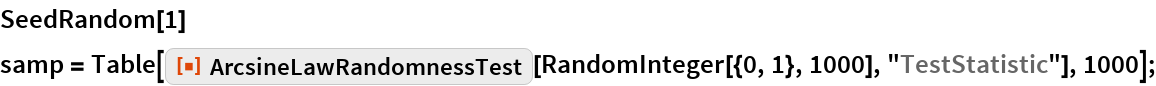In:=Out=